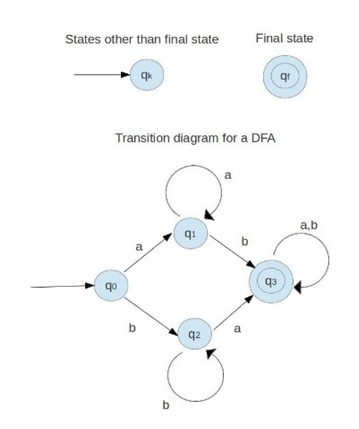# Introduction to Theory of Computation (TOC)

Theory of computation: Here, we are going to learn about the introduction of theory of computer, its definition, what is automata, its example, applications etc.
Submitted by Mahak Jain, on November 11, 2018

Definition:

It is a branch of computer science that actually finds out how a problem could be solved efficiently with the help of a model of computation using some kind of an algorithm.

What is an automata?

It is an abstract model of digital computer. There is input tape in every automata to read input written on a file one cell at a time from left to right. Some form of output is also produced by the automation. There is also a control unit which can be in any one of the finite no of internal states.

Examples of automata machines:

Finite state machine, context free language, Turing machine, etc.

Practical applications of theory of computation:

• Traffic lights
• Lifts and elevators
• Marketing
• Compilers
• Vending machines
• Cloud computing

Prerequisites:

• Symbol: a, b, c, 0, 1, 2, ...
• Alphabet: finite collection of symbols {a,b}, {0,1,2} , ...
• String: sequence of symbols aaaaa,ababa,…..
• Length of string: no. of symbols in a string |aaabc|=5
• Language: set of strings {aaaabbb,abbbc}, ...
• Powers of 'Σ': If Σ = {c,b} then
Σ0 = Set of all the strings over Σ of length 0. {ε}
Σ1 = Set of all the strings over Σ of length 1. {c, b}
Σ2 = Set of allthe strings over Σ of length 2. {cc, cb, bc, bb}
i.e. 2|= 4 and Similarly, |Σ3| = 8
• Cardinality: it is the number of the elements in the set.
• Transition function: An automata is supposed to operate in a discrete time frame.at one point of time, the control unit is in some internal state and the input mechanism is scanning a certain symbol on the input tape. The internal state of this control unit at the next point of time or step is called the next state or the transition function. This transition function gives the next state in terms of the current state, the current input symbol on the input tape, and the information currently in the temporary storage. During the transition from the one step to the next step, the output may get generated or the information in the temporary storage may get changed.
• Move: The term configuration refers to a particular state of the control unit, the input tape, and the temporary storage. The transition from one stage to the next is called a move.

What is a transition graph?

It is a directed graph. There is an initial state, internal states, and final states. the final state is also known as an accepting state. The vertices denoted by circles are used to represent states. The final state is denoted by two circles. The initial state has a one side arrow pointed towards it attached. The edges are the lines with the arrows which show the transition or the move. The edge connects one state to the other state. The edge may also be a self-loop pointing towards itself. The state is changed by using a state function and an output is given using a machine function.

Ex: